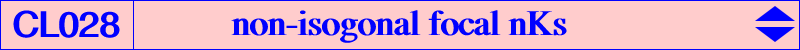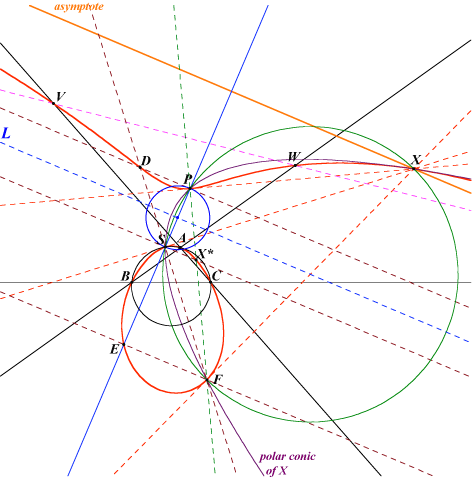Given a pole W of isoconjugation which is not the Lemoine point K, there is one and only one focal (circular) nK with pole W. Let L be the W-isoconjugate of the circumcircle i.e. the trilinear polar of the isotomic conjugate of the isogonal conjugate of W. Denote by : S the trilinear pole of the line WK (on the circumcircle) and S* its W-isoconjugate (on the line at infinity), P the reflection of S in L and F = P* its W-isoconjugate, R the orthocorrespondent of P, X* the intersection of the lines SS*, PP* and X its W-isoconjugate, antipode of F on the circle PSF.R is the root of the focal cubic and the trilinear polar of R meets the sidelines of ABC at U, V, W on the cubic. F is the singular focus and X the intersection of the cubic with its real asymptote, the homothetic of L under h(F,2). The circle with diameter FX passes through P and S. Thus, the lines PF, PX and SF, SX are perpendicular. The cubic passes through the orthogonal projection E of F on the line SP and D intersection of the lines FS, PS*. D and E are isoconjugate. The tangents at P, S, F and the real asymptote concur at X hence the polar conic of X is the hyperbola passing through P, S, F, S* and X. The polar conic of F is the circle passing through F, centered on PS and orthogonal to the circle with diameter PS. In other words, its center is the intersection of PS with the perpendicular at F to FX.Locus properties : This cubic is the locus of M such that the circle with diameter MM* passes through P. A circle with center Z on L passing through P and S intersects the line ZF at M, N on the cubic. The lines MM*, NN* intersect on the perpendicular at S to PS and the following triads of points are collinear on the cubic : F, M, N - F, M*, N* - P, M, N* - P, M*, N. The contacts M, N of the tangents drawn from F to a circle centered on PS and orthogonal to the circle with diameter PS are two points on the cubic. Moreover, the lines MM* and NN* intersect on DE and the following triads of points are collinear on the cubic : X, M, N - X, M*, N* - X*, M*, N - X*, M, N*.Other properties : The most remarkable example of such cubic is K091, the only isotomic focal nK. This cubic is a nK0 if and only if W lies on K214. When W lies on the orthic axis, this cubic decomposes into a rectangular circum-hyperbola (P = H) and the line at infinity. When W lies on the circum-conic with perspector X(184), this cubic decomposes into a line through O (P* = O) and the circum-circle. This circum-conic contains X(112), X(248), X(906), X(1415), X(1576)... This cubic is an isogonal focal pK with respect to the triangle PSF, the pivot being S*. This shows that is must contains the in/excenters of triangle PSF which are the centers of anallagmaty of the curve. These points obviously lie on the rectangular hyperbola which is the polar conic of S*. Its center is the second intersection of the line XS* with the circle PSF. The asymptotes are therefore the real asymptote of the cubic and the parallel at F to PS. Any circle with center F meets the cubic at two points collinear with X.The table gives a short selection of non-isogonal focal nKs (the red point is the singular focus).Pole Centers on the cubic Cubic / remarks X(2) X(67), X(99), X(316), X(523) K091 = nK(X2, X11056, X67) X(44) X(8), X(100), X(900), X(1319) nK(X44, X1997, X8) X(50) X(3), X(110), X(186), X(526) K933 = nK(X50, X1993, X3) X(67) X(2), X(67), X(525), X(935) X(111) X(6), X(523), X(671), X(691) X(187) X(2), X(110), X(187), X(690) nK(X187, X1992, X2) X(385) X(76), X(99), X(804), X(1691) X(524) X(69), X(99), X(468), X(690) X(1989) X(4), X(265), X(476), X(523) K1280 = nK(X1989, X18883, X4) X(2161) X(1), X(80), X(522), X(2222) nK(X2161, X2006, X1) X(2183) X(8), X(109), X(1457), X(2804)